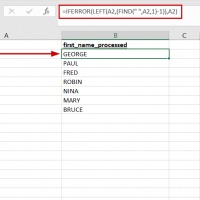## EXCEL: How to extract text before a comma, space or period [SOLVED]Excel tip:  If you try to extract text before a space ” “, comma “,” or period “.” in Excel you may try something along these lines: =LEFT(A2,(FIND(” “,A2,1)-1)) However, if you have non-matching records, i.e. something without a space in the previous example then you will get the following result: #VALUE! Since that is probably not what you wanted, try the following: =IFERROR(LEFT(A2,(FIND(” “,A2,1)-1)),A2) Which will result in either (A) the shortened result (where applicable) or (B) the original